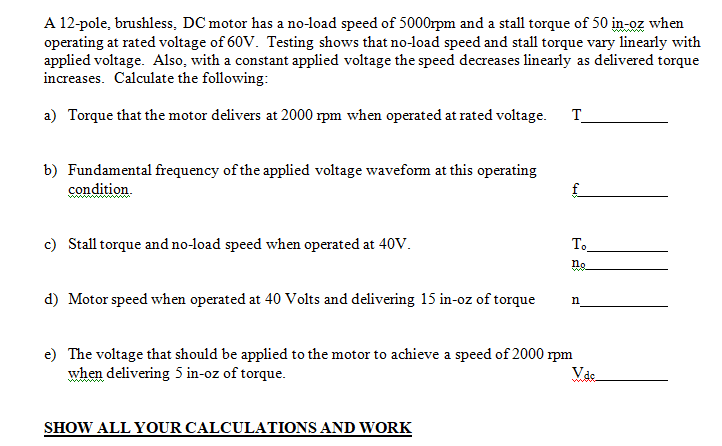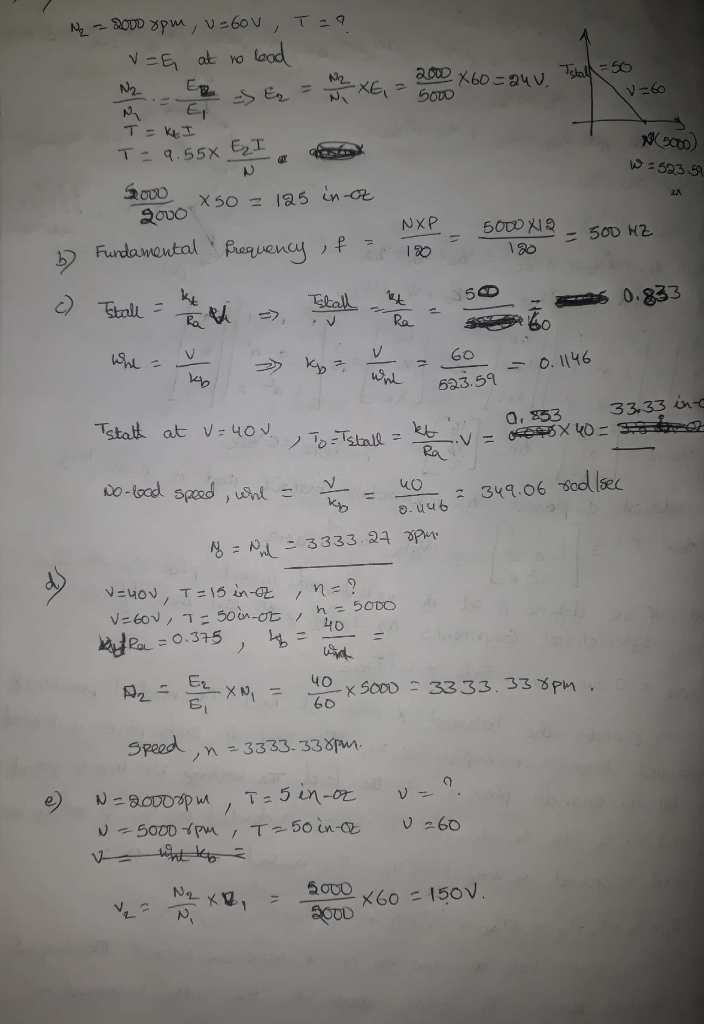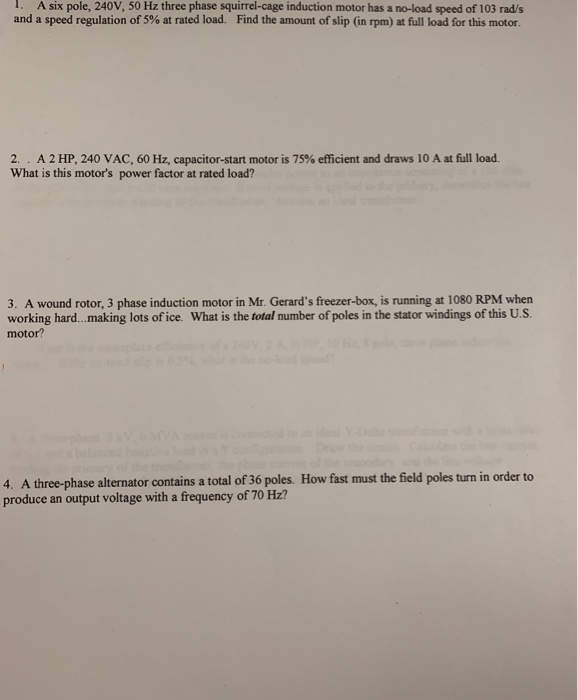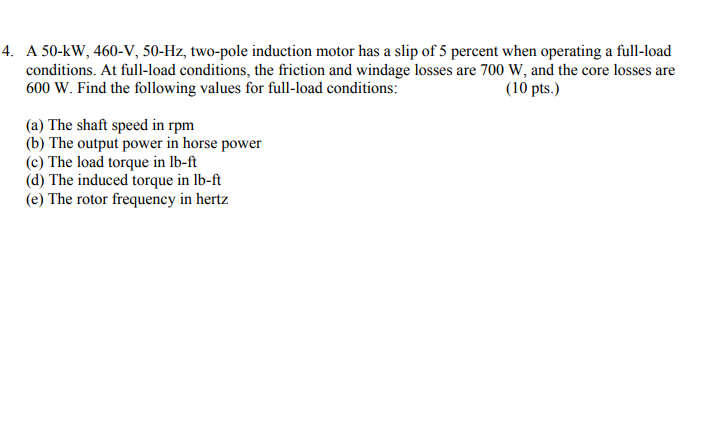Question#### Earn Coins

Coins can be redeemed for fabulous gifts.

Similar Homework Help Questions
• ### A 3-phase 50Hz 2-pole 440V (L-L rms) induction motor drive has a rated speed of 2955...

A 3-phase 50Hz 2-pole 440V (L-L rms) induction motor drive has a rated speed of 2955 rpm and rated torque of 35 Nm. This motor drives a load with a torque characteristic that increases linearly with speed such that it equals the load torque at the rated speed. It draws a current of 2.1 A(rms) per phase at the rated voltage at a power factor of 0.81 (lagging). The stator resistance Rs = 2.0 ohms. At the rated operating speed,...

• ### A three-phase, 50-Hz, 4-pole, 575-V (line-line, rms) induction motor drive has a full-load (rated) speed of...

A three-phase, 50-Hz, 4-pole, 575-V (line-line, rms) induction motor drive has a full-load (rated) speed of 1450 rpm. The rated torque is 50 Nm. Keeping the air gap flux-density peak constant at its rated value, (a) plot the torque-speed characteristics (the linear portion) for the following values of the frequency: 50 Hz, 40 Hz, 30 Hz and 20 Hz. (b) This motor is supplying a load whose torque demand increases linearly with speed such that it equals the rated motor...

• ### 1. A six pole, 240V, 50 Hz three phase squirrel-cage induction motor has a no-load speed...1. A six pole, 240V, 50 Hz three phase squirrel-cage induction motor has a no-load speed of 103 rad/s and a speed regulation of 5% at rated load. Find the amount of slip (in rpm) at full load for this motor. 2.. A 2 HP, 240 VAC, 60 Hz, capacitor-start motor is 75% efficient and draws 10 A at full load. What is this motor's power factor at rated load? 3. A wound rotor, 3 phase induction motor in Mr....

• ### 4. A 50-kW, 460-V, 50-Hz, two-pole induction motor has a slip of 5 percent when operating...4. A 50-kW, 460-V, 50-Hz, two-pole induction motor has a slip of 5 percent when operating a full-load conditions. At full-load conditions, the friction and windage losses are 700 W, and the core losses are 600 W. Find the following values for full-load conditions: (10 pts.) (a) The shaft speed in rpm (b) The output power in horse power (c) The load torque in lb-ft (d) The induced torque in lb-ft (e) The rotor frequency in hertz